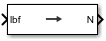Force Conversion

Convert from force units to desired force units

• Library:
• Aerospace Blockset / Utilities / Unit Conversions

•Description

The Force Conversion block computes the conversion factor from specified input force units to specified output force units and applies the conversion factor to the input signal.

The Force Conversion block port labels change based on the input and output units selected from the Initial unit and the Final unit lists.

Ports

Input

expand all

Force, specified as a scalar or array, in initial force units.

Dependencies

The input port label depends on the Initial unit setting.

Data Types: double

Output

expand all

Force, returned as a scalar or array, in final force units.

Dependencies

The output port label depends on the Final unit setting.

Data Types: double

Parameters

expand all

Input units, specified as:

 lbf Pound force N Newtons

Dependencies

The input port label depends on the Initial unit setting.

Programmatic Use

 Block Parameter: IU Type: character vector Values: lbf | N Default: lbf

Output units, specified as:

 lbf Pound force N Newtons

Dependencies

The output port label depends on the Final unit setting.

Programmatic Use

 Block Parameter: OU Type: character vector Values: lbf | N Default: N

Extended Capabilities

C/C++ Code GenerationGenerate C and C++ code using Simulink® Coder™.

Introduced before R2006a

Aerospace Blockset DocumentationGet trial now